Mplus
Sunday
September 24, 2023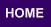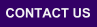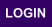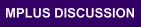Mplus Mplus at a GlanceGeneral DescriptionMplus Programs Pricing Version History System Requirements Platforms
 Analyses/ResearchMplus Examples Papers References
 Search

New Mplus Book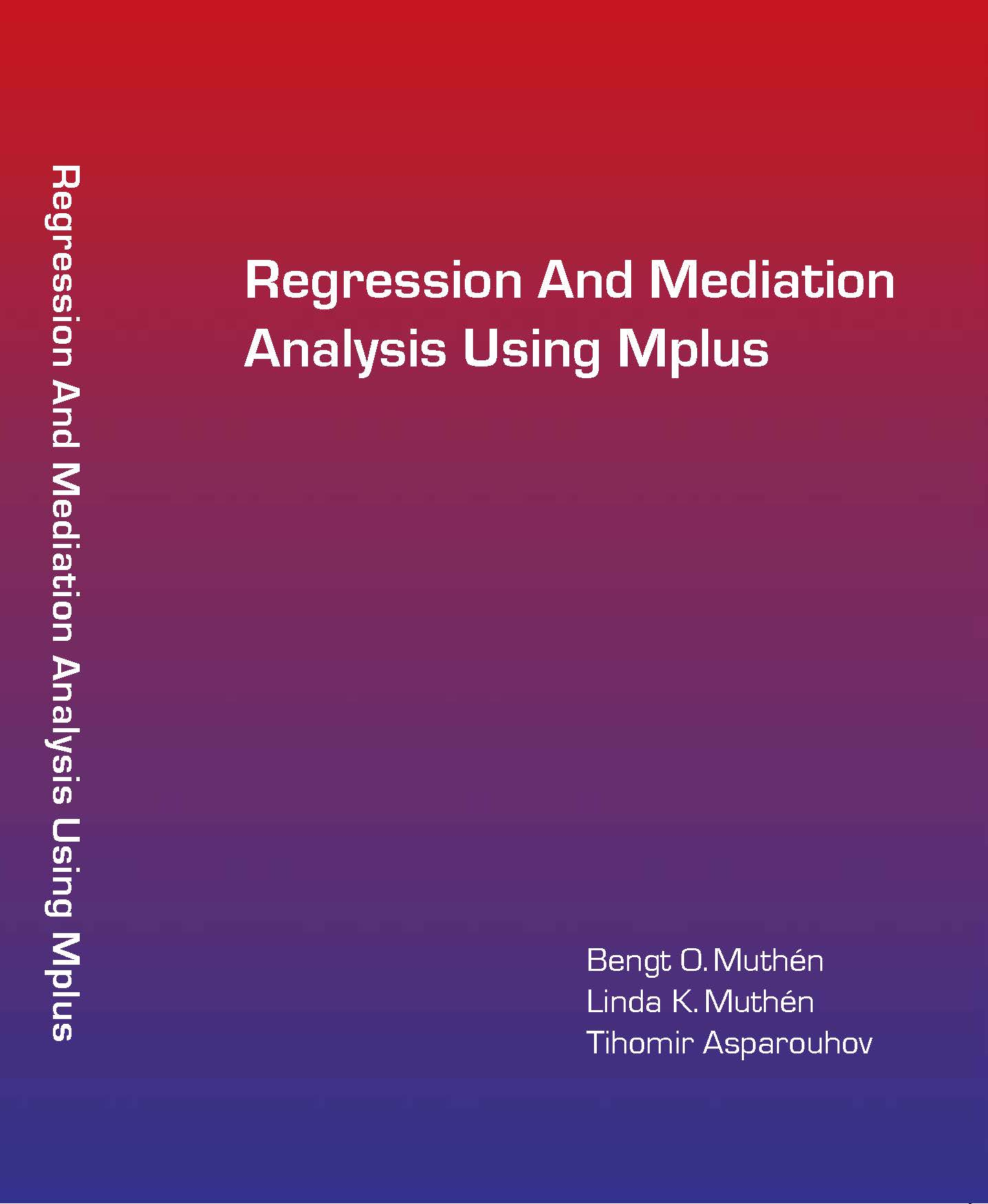Regression And Mediation Analysis Using Mplus

Bengt O. Muthén, Linda K. Muthén, Tihomir Asparouhov

The inspiration to write this book came from many years of teaching about Mplus and answering questions on Mplus Discussion and Mplus support. It became clear that once people leave school, it is difficult to keep up with the newest methodology. The purpose of this book is to provide researchers with information that is not readily available to them and that we believe is important for their research. Many topics such as linear regression analysis; mediation analysis; causal inference; regression analysis with categorical, count, and censored outcomes; Bayesian analysis; and missing data analysis have entire books devoted to them. This book does not attempt to replace these books but rather to give useful and manageable summaries of these topics and show how the analyses are implemented in Mplus. The technical level is kept at a minimum but still requires an introductory statistics background and a good background in regression.

Chapter 1 covers linear regression analysis including regression with an interaction, multiple-group analysis, missing data on covariates, and heteroscedasticity modeling. Chapter 2 covers mediation analysis with a continuous mediator and a continuous outcome including moderated mediation. Chapter 3 covers special topics in mediation analysis that are not normally found in books on mediation analysis. These include Monte Carlo simulation studies of mediation and moderated mediation, model misspecification due to omitted variables and confounders, instrumental variable estimation, sensitivity analysis, multiple group analysis of moderated mediation, and measurement error. Chapter 4 covers causal inference based on counterfactuals for mediation analysis with a continuous mediator and a continuous outcome. Chapter 5 covers regression analysis for categorical dependent variables including binary, ordinal, and nominal variables. Chapter 6 covers regression analysis for a count dependent variable including the following models: Poisson, Poisson with a random intercept, zero-inflated Poisson, negative binomial, zero-inflated negative binomial, two-part (hurdle) with zero-truncation, and varying-exposure. Chapter 7 covers regression analysis for a censored dependent variable including the following models: censored-normal (tobit), censored-inflated, sample selection (Heckman), two-part, and switching regressions. Chapter 8 covers causal inference for mediation analysis with a binary outcome and a continuous mediator, a count outcome and a continuous mediator, a two- part outcome and a continuous mediator, a binary and an ordinal mediator, a nominal mediator, and a mediator with measurement error. Chapter 9 discusses Bayesian analysis and uses it to estimate several mediation examples which show how it can be used as an alternative to maximum likelihood estimation. Chapter 10 discusses several approaches to missing data modelling including missing completely at random (MCAR), missing at random (MAR), and not missing at random (NMAR) including selection modeling.

Product Details

Paperback: 519 pages
Publisher: Muthén & Muthén
Language: English
ISBN 978-0-9829983-1-1
Product dimensions: 9.5 x 7.25 x 1

To Order

The book can be ordered from the online store.

Web Page for Examples

Mplus inputs and outputs for the book examples are posted along with data sets where available. A total of 140 analyses are shown.

Errata

Errata for first and second printing.

• Preface
1. Linear regression analysis
1. Linear regression analysis assumptions
2. Linear regression estimation
1. Residuals
2. Outliers
3. Linear regression R-square
4. Linear regression standardization
5. Example: Regression with one covariate
1. Individual residuals and outliers
2. Reporting results
6. Multiple covariates
1. Linear regression with two continuous covariates
Interaction between two continuous covariates
2. Linear regression with one binary and one continuous covariate
Interaction between a binary and a continuous covariate
7. Example: Regression with two covariates
1. Reporting results
8. Example: Regression with an interaction
1. Reporting results
Presenting parameter estimates
Presenting results graphically
9. Special topics
1. Standardized coefficients greater than one
2. Standardized coefficients differing in significance from unstandardized coefficients
3. Two-group regression analysis
Example: Two-group regression analysis of an intervention study
4. Bringing covariates into the model
Missing data on x
Example: Bringing a covariate into the model for the intervention example using a two-group analysis
5. The Bayesian Information Criterion (BIC) and the Akaike Information Criterion (AIC)
6. Heteroscedasticity modeling
Example: Heteroscedasticity modeling of LSAY math data
7. Random coefficient regression
Example: Random coefficient regression for LSAY math data
2. Mediation analysis
1. A prototypical mediation model
2. Mediation modeling techniques
1. Estimation
Indirect effect standard errors and confidence intervals
2. Standardization for mediation models
3. Model testing
3. Example: Sex discrimination
1. Inspecting the data and reporting results
1. Reporting results
2. The saturated model
5. Multiple mediators
1. Example: Parallel mediators for media influence
2. Example: Sequential mediators of socioeconomic status
6. Moderated mediation
1. Case 1 (xz): Regression of y on x, m on x, both moderated by z
2. Case 2 (mz): Regression of y on m moderated by z
3. Case 3 (mx): Regression of y on m moderated by x
4. Combined moderation case
5. Example: Case 1 moderated mediation in an intervention of aggressive behavior in the classroom
Testing significance of effects at specific moderator values
Creating a plot with bootstrap confidence intervals for the effects at a range of moderator values
Combination of significance of effect at specific moderator values and plot of confidence intervals using MODEL INDIRECT
6. Example: Case 2 moderated mediation for work team behavior
7. Example: Case 3 moderated mediation of simulated data
8. Example: Combined moderated mediation for sex discrimination
3. Special topics in mediation analysis
1. Monte Carlo simulation study of mediation
1. Example: Monte Carlo study of indirect effects
2. Monte Carlo studies of moderation
1. Example: Moderation of the regression of m on x
2. Example: Moderation of the regression of y on m
3. Model misspecification
1. Example: Omitted moderator
2. Example: Omitted mediators
3. Example: Confounders
4. Instrumental variable estimation
1. Example: IV estimation with mediator-outcome confounding
Bias and coverage of IV estimation of the indirect effect
Comparing IV and ML standard errors and power
IV estimation dependence on the size of the x, m correlation
Comparison of IV and maximum-likelihood estima- tion when the assumptions behind both approaches are violated
5. Sensitivity analysis
1. Example: Sensitivity analysis for an experimental study of sex discrimination in the workplace
2. Example: Sensitivity analysis in a Monte Carlo study
6. Multiple-group mediation analysis
1. Relating multiple-group parameters to interaction parameters
2. Modification indices
3. Example: Two-group analysis of moderated mediation for sex discrimination
7. Measurement errors and latent variables
1. Measurement error in an independent variable
2. Measurement error in a mediator
3. Example: Monte Carlo simulation study of measurement error in the mediator
4. Known reliability
5. Multiple indicators
Reliability of a sum of indicators
Structural equation modeling with a factor analysis measurement model
4. Causal inference for mediation
1. Causal assumptions
2. Potential outcomes and counterfactuals
1. Example: Hypothetical potential outcome data
3. Basics of counterfactually-defined effects
1. Example: Hypothetical mediation potential outcomes
4. Direct and indirect effects
1. Direct effects
2. Indirect effects
3. Total effect decomposition
4. Example: Hypothetical mediation data analysis
5. Causal effect formulas
1. Example: Effects in the simple mediation case
2. Example: Effects with moderation of Y regressed on M (case 3)
3. Example: Effects in the combined moderation case
4. Example: Effects combining case 1 and case 2 moderation
5. Multiple mediators
6. Summary
5. Categorical dependent variable
1. Basic concepts for categorical variables
1. Binary variables
2. Binary dependent variable
1. Example: OLS, logistic, and probit regression of coal miner respiratory problems
2. Modeling with a logistic regression function
3. Modeling with a probit regression function
4. Estimation of the logistic and probit regressions
5. Probability curve formulation versus a latent response variable formulation
6. R2 and standardization
R2 for a binary outcome
Standardization
7. Example: Logistic and probit regression of British coal miner data
Logistic regression
Probit regression
Computation of estimated probabilities
Comparing logistic and probit regression coefficients
Comparing the logistic and probit regression models by BIC
8. Logistic and probit regression with one binary and one continuous x
9. Logistic regression and adjusted odds ratios
10. Example: Adjusted odds ratios for alcohol survey data
11. Example: Adjusted odds ratios for educational achievement data
3. Ordinal dependent variable
1. Probability curve formulation of ordinal dependent variable regression
2. Latent response variable formulation of ordinal dependent variable regression
3. Example: Sample probits for drinking related to age and income
4. Testing the parallel probability curve assumption behind the ordinal regression model
5. Example: Ordinal logistic regression of mental impairment
Estimated odds ratio
Estimated probabilities
Odds ratio with an interaction
4. Nominal dependent variable
1. Example: Multinomial logistic regression of antisocial behavior
6. Count dependent variable
1. Poisson model
2. Poisson model with a random intercept
3. Zero-inflated Poisson model
4. Negative binomial model
5. Zero-inflated negative binomial model
6. Two-part (hurdle) model with zero-truncation
7. Varying-exposure model
8. Comparing models
9. Example: Count regression of marital affairs
1. Poisson, Poisson with a random intercept, and negative binomial models
Negative binomial model
2. Zero-inflated Poisson and zero-inflated negative binomial models
3. Two-part (hurdle) modeling
4. Conclusion for marital affairs analyses
10. Example: Poisson with varying exposure
7. Censored dependent variable
1. Basic concepts for a censored variable
2. Censored-normal (tobit) regression
3. Censored-inflated regression
4. Sample selection (Heckman) regression
1. Example: Simulated sample selection data
5. Two-part regression
6. Example: Methods comparison on alcohol data
1. Analysis results for the four models
Loglikelihood and BIC comparisons of the four models
Comparing the results for the censored-normal (tobit) and censored-inflated models
Comparing the results for the sample selection (Heckman) and two-part models
Comparing the results for the censored-inflated and two-part models
Comparing the fit for estimated probabilities and means for the censored-inflated and two-part models
7. Switching regressions
1. Example: Monte Carlo simulation of switching regressions
8. Mediation non-continuous variables
1. Binary outcome, continuous mediator
1. A simple hypothetical mediation model
2. Total, indirect, and direct effects in terms of differences in probabilities
3. Causal effect formulas for a continuous M and a binary Y
4. Causal effect formulas applied to a simple mediation model with a binary outcome and a continuous mediator
5. Causal effect formulas defined on the odds ratio scale
Odds ratio effects assuming a rare outcome
6. Example: Intention to use cigarettes
Probit regression
Logistic regression
7. Example: HPV vaccination trial
No intervention-mediator interaction
Intervention-mediator interaction
Analysis results
2. Count outcome, continuous mediator
1. Causal effect formulas for a count outcome
2. Example: Aggressive behavior and school removal
Estimated count probabilities
3. Two-part outcome, continuous mediator
1. Causal effect formulas for a two-part outcome
2. Example: Two-part mediation analysis of economic stress data
Causal effects for two-part modeling
Causal effects for regular modeling with log y
Causal effects for regular modeling without log y
4. Binary and ordinal mediator
1. Causal effect formulas for a binary mediator
2. Ordinal mediator
3. Latent response variable mediator
4. Estimation
5. Example: Hypothetical data from the potential outcome example with a binary mediator and a continuous outcome
6. Example: Ordinal mediator for intention to use cigarettes
7. Example: Pearls artificial 2 x 2 x 2 example
5. Nominal mediator
1. Causal effect formulas for a nominal mediator
2. Estimation
3. Example: Hypothetical data with a nominal mediator and a binary outcome
6. Mediator with measurement error
1. Example: A Monte Carlo simulation study for a mediator measured with error
2. Example: An intervention study of aggressive behavior in the classroom and juvenile court record
9. Bayesian analysis
1. Prior, likelihood, and posterior
1. Posterior distribution for the mean of a normal distribution
2. Types of priors
3. Non-normality of parameter distributions
2. Markov Chain Monte Carlo (MCMC)
1. Example: Bayesian estimation of a mean with missing data for LSAY math
Input for Bayesian analysis
2. Plots
Trace plot
Autocorrelation plot
Posterior distribution plot
3. Convergence checking
3. Model fit
4. Bayes versus ML intervals
5. Example: Mediation model for media influence
The Potential Scale Reduction (PSR) convergence criterion
Trace plot
Autocorrelation plot
Inspecting model fit and parameter estimates
6. Example: Mediation model for firefighter data
1. Non-informative priors
2. Informative priors
7. Example: Model testing of direct effects
8. Example: High school dropout and missing data
1. Missing data on the mediator (n = 2, 213)
Bayesian analysis
Maximum-likelihood analysis
2. Missing data on the control variables (n = 2, 898)
Assuming normality for all covariates
Acknowledging that some control variables are binary
10. Missing data
1. Example: Missing data information
2. MCAR, MAR, and NMAR
1. MCAR: Missing completely at random
2. MAR: Missing at random
3. NMAR: Not missing at random
3. MAR for bivariate normal variables (H1 case)
1. Listwise versus ML
2. Maximum-likelihood estimation in the bivariate case with missing on one variable
3. The EM algorithm
4. Multiple imputation
5. Example: Estimating sample statistics for intervention data
4. MAR for regression (H0 case)
1. Missing data and selection on x or y
2. Regression analysis with missing data
3. Technical aspects of ML assuming MAR
4. Example: MAR simulated data analysis
5. Missing data correlates
Example: Simulation study with missing data correlate of missing on y
5. NMAR
1. Example: Simulated NMAR data with missing influenced by the latent outcome
2. Example: Selection modeling versus ML assuming MAR when MAR holds
6. Example: Comparing missing data methods
7. Missing data on covariates
1. Example: Simulation study of missing on binary covariates
Inputs for generating and analyzing data assuming MAR
Inputs for generating and analyzing data under NMAR
Simulation results

Appendices

• A Covariance algebra
• A.1 Definition of expectation
• A.1.1 Rules for expectation
• A.2 Definition of covariance and variance
• A.2.1 Rules for variance
• A.3 Functions of random variables
• A.4 Example: Covariance algebra rules applied to linear regression
• A.5 Example: Derivation of the slope attenuation
• A.6 Example: Variance of a variable measured with error